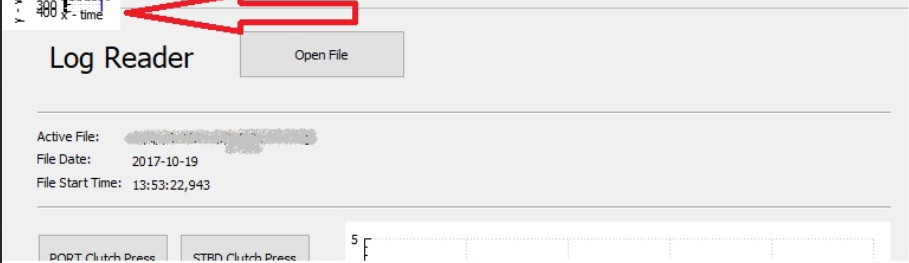• Hello again!

I'm having trouble with the following code:

``````void MainWindow::graphSelected(QStringList _graphingData) {
QList<QStringList> list;
QList<double> data;
foreach (const QString &s, _graphingData) {
const QStringList parts = s.split(",");
list.append(parts);
Q_ASSERT(parts.size() >= 3);
data.append(parts.at(2).toDouble());
}

QVector<double> y = QVector<double>::fromList(data);

if (Debug) {
qDebug() << list;
qDebug() << data;
}

int j = data.size();
QVector<double> x[j];

for (int i=0; i<j; i++) {
x[i]<<i;
}
customPlot->graph(0)->addData(x, y);  // <- error "no matching function for call to 'QCPGraph::addData(QVector<double> [j], QVector<double>&)'
customPlot->xAxis->setLabel("x - time");
customPlot->yAxis->setLabel("y - value");
customPlot->graph(0)->rescaleAxes();
customPlot->replot();
}
``````

My understanding is that addData is supposed to take (QVector<T>, QVector<T>). I've spent hours trying to solve this - different data types, different ways of calling it (->setData...), with no luck, so I'm asking for help (again).

Thanks.

• Hi @MScottM,

The problem, I suspect, is this line:

QVector<double> x[j];

Here you have an array of `j` vectors of `0` doubles each.

``````QVector<double> x(j);
``````

Which gives a single vector of `j` doubles.

Cheers.

• Once again @Paul-Colby, that did the trick! That and changing x[i]<<i; back to x[i]=i; I knew it was some kind of rookie mistake.

It seems to be plotting now, but instead of putting the data on the QWidget that I promoted to a QCustomPlot, it is squishing it up in the corner of my main window...Have you or anyone seen this behavior, or I could take this to the QCustomPlot support forum...?

Thanks again for putting me on the right track!

• Hi
Are you sure that is the promoted one ?
From code you say
customPlot->xxxx
for a promoted its normally
ui->customPlot->xxxx

It seems more like one you NEW and not assign to a layout.

• Aha! That was it @mrjj. In my MainWindow setup I had: ui->customPlot = new QCustomPlot(this); I probably put it in there while following some tutorial or other on QCustomPlot.

Solved!